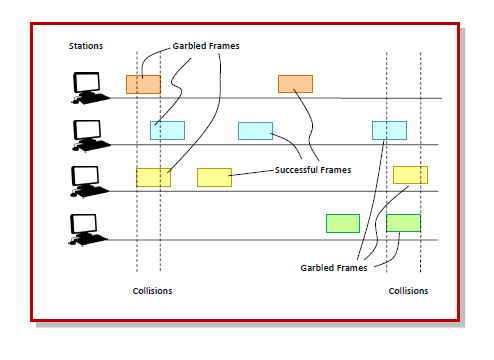# Slotted ALOHA

ALOHA is a medium access control (MAC) protocol for transmission of data via ashared network channel. Using this protocol, several data streams originating from multiple nodes are transferred through a multi-point transmission channel. There are two types of ALOHA protocols – Pure ALOHA and Slotted ALOHA.

Slotted ALOHA was introduced in 1972 by Robert as an improvement over pure ALOHA. Here, time is divided into discrete intervals called slots, corresponding to a frame.

Working Principle

The communicating stations agree upon the slot boundaries. Any station can send only one frame at each slot. Also, the stations cannot transmit at any time whenever a frame is available. They should wait for the beginning of the next slot.

However, there still can be collisions. If more than one frame transmits at the beginning of a slot, collisions occur. The collision duration is 1 slot. The situation is depicted in the following diagram−Throughput of Slotted ALOHA

Let T be the frame time, i.e. the time required for 1 frame to be transmitted.

Let G be the number of transmission attempts per frame time.

The probability that 𝑘 frames are generated during the frame time is given by the Poisson distribution−

$$P(k)=\frac{G^k e^{-G}}{k!}$$

From this we can say that the probability that 0 frames are generated ( 𝑘 = 0 ) during the frame time is $e^{-G}$.

In case of slotted ALOHA, the vulnerable time period for collision between two frames is equal to time duration of 1 slot, which is equal to 1 frame time, i.e. 𝑇.In 𝑇 time, average number of transmission attempts is G.

The probability that 0 frames are initiated in the vulnerable time period will be−

$$P(0) = e^{-G}$$

The throughput, 𝑆, is calculated as the number of transmission attempts per frame time, 𝐺, multiplied by the probability of success, 𝑃P(0).

$$S = G.P(0)$$

or $$S = Ge^{-G}$$

Maximum Throughput of Slotted ALOHA

The maximum throughput occurs when G = 1.

The maximum throughput is thus−

$$S_{max}=1\times e^{-1}=\frac{1}{e}=0.368$$

Thus, it can be seen that the maximum throughput is 36.8% in slotted ALOHA,which is an improvement over maximum throughput of 18.4% in pure ALOHA.